## 2. 5. 2 Constants and Second Order Functions

In order to provide the use of simple numerical constants, constant functions are introduced which return a constant value independently from the actual argument.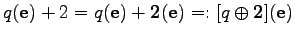(2.37)

It is obvious that the numerical value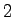is structurally different from the constant function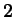. However, the final formalism has to be defined in a programming language and, therefore, a discrimination ofandwould lead to an unnecessary complication of the notation. In analogy to the basis operator symbols, the structure of the given functional entities is clear and depends on the context in which it is used.

Next, function application is introduced in the following way, where the functional part is strictly separated from the argument part.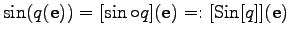(2.38)

The application of the function sin() on the evaluation term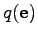may also be interpreted as the application of the compound function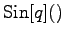on the element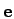. In the following the functions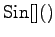and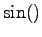have a different meaning. Whereas sin() is the typical sine function which is applied on numerical values and results in a numerical value, the second-order function Sin[]() is a function which is applied to a functional expression. Again, it can be determined from the context, if sin() or Sin[]() is meant.

Large expressions can be formed from the base operations. These operations have in common that all quantities which are employed are implicitly evaluated on the common element of evaluation, for instance a vertex, an edge or a cell. In the following section, methods are introduced which perform operations on topologically different elements while using traversal mechanisms as described in Section 2.3.

Michael 2008-01-16